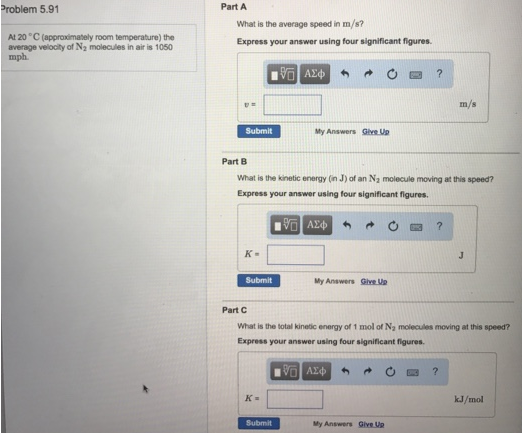# Problem: At 20°C (approximately room temperature) the average velocity of N 2 molecules in air is 1050 mph.Part A. What is the average speed in m/s? Express your answer using four significant figures.Part B. What is the kinetic energy (in J) of an N 2 molecule moving at this speed? Express your answer using four significant figures. Part C. What is the total kinetic energy of 1 mol of N 2 molecules moving at this speed? Express your answer using four significant figures.

###### FREE Expert Solution
83% (115 ratings)###### Problem Details

At 20°C (approximately room temperature) the average velocity of N 2 molecules in air is 1050 mph.

Part A. What is the average speed in m/s? Express your answer using four significant figures.

Part B. What is the kinetic energy (in J) of an N 2 molecule moving at this speed? Express your answer using four significant figures.

Part C. What is the total kinetic energy of 1 mol of N 2 molecules moving at this speed? Express your answer using four significant figures.